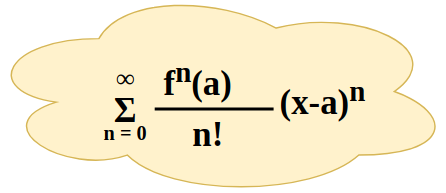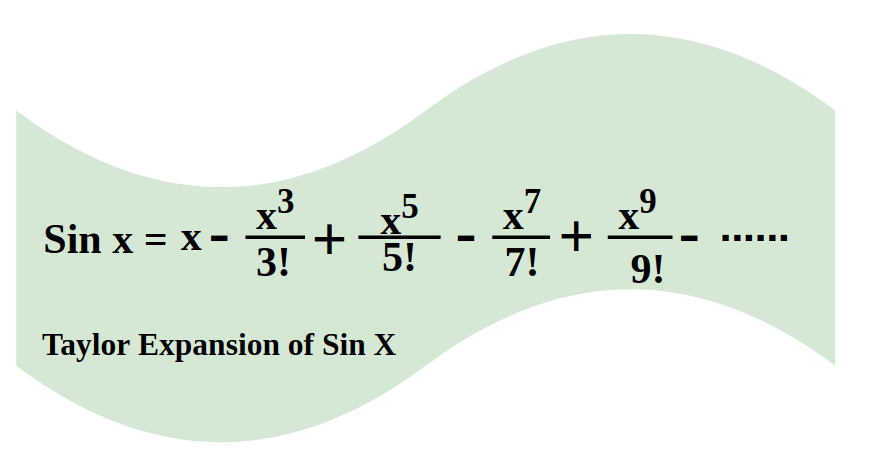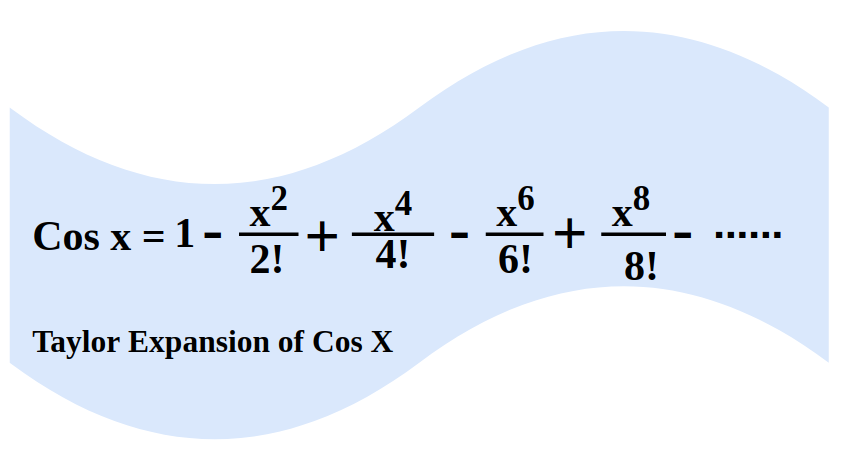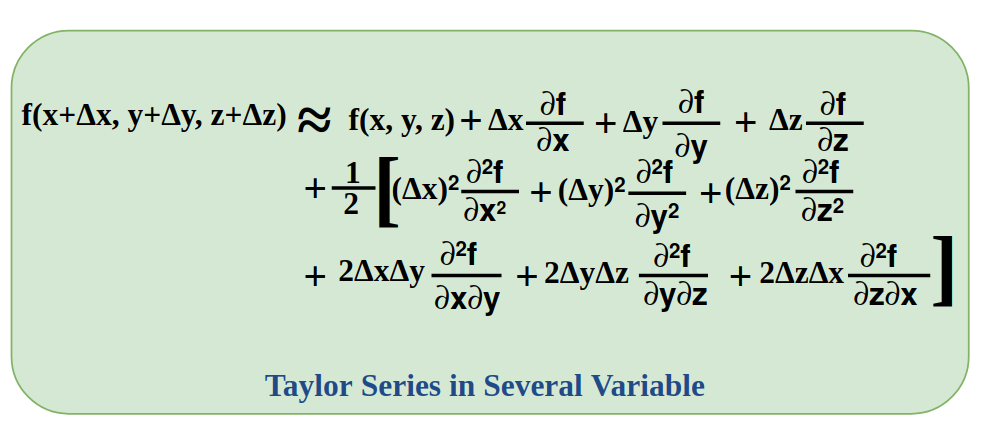# Taylor Series

Taylor Series is the series which is used to find the value of a function. It is the series of polynomials or any function and it contains the sum of infinite terms. Each successive term in the Taylor series expansion has a larger exponent or a higher degree term than the preceding term. We take the sum of the initial four, and five terms to find the approximate value of the function but we can always take more terms to get the precise value of the function.

## Taylor Series Expansion

Taylor series expansion of the real and composite function f(x) whose differentiation exists in a close neighbourhood is,

f(x) = f(a) + [f'(a)’/1!](x-a) + [f”(a)/2!](x-a)2 + [f”'(a)/3!](x-a)3 +…

Taylor Series in terms of sigma notation is,## Taylor Series & Maclaurin Series

The above Taylor series expansion is given for a real values function f(x) where f’(a), f’’(a), f’’’(a), etc., denotes the derivative of the function at point a. If the value of point ‘a’ is zero, then the Taylor series is also called the Maclaurin series.

## Taylor’s Series Formula

Taylor’s series formula is used to find the value of any function around the particular value. Suppose we have to find the value of the real and composite function f(x) at point a, such that the differentiation of the function is defined in the closed neighbourhood of the function then the function is represented by the formula,

f(x) = f(a) + [f'(a)’/1!](x-a) + [f”(a)/2!](x-a)2 + [f”'(a)/3!](x-a)3 +…+ f(n)/n!(x-a)n

where,
f(x) is the real or complex value function that is infinitely differentiable
n is the number of times the function is differentiated
f(n) is the n derivative of the function f(x)

## Taylor Series Theorem Proof

The statement for the Taylor Series Theorem is

Statement: For a real or complex-valued function f(x) which is differentiable on the neighbourhood of a number the Taylor series is,

f(x) = f(a) + [f'(a)’/1!](x-a) + [f”(a)/2!](x-a)2 + [f”'(a)/3!](x-a)3 +…+ f(n)(x)/n!(x-a)n

Proof:

We know that power series is defined as,

f(x) = ∑ anxn = a0 + a1x + a2x2 + a3x3 + …            (i)

where, 0≤n<∞

for x = 0

f(x) = ao

The differentiation of the function f(x) from eq (i) is,

f'(x) = a1 + 2a2x + 3a3x2 + 4a4x3 …             (ii)

substituting x = 0 in f'(x)

f'(0) = a1

Differentiating eq (ii) again,

f”(x) = 2a2 + 6a3x + 12a4x2 + …

substituting x = 0 in f”(x)

f”(0) = 2a2

f”(0)/2! = 2a2/2 = a2

similarly, f(n)(0)/n! = an

Now substituting all these values in eq(i)

f(x) = f(0) + f'(0)x + f”(0)/2!(x)2 + f”'(0)/3!x3 + …

Generalising the function f(x) we get,

f(x) = b + b1(x-a) + b2(x-a)2 + b3(x-a)3 + …

Now taking x =a,

bn = f(n)(a)/n!

Substituting the value of bn in a generalised form of f(x)

f(x) = f(a) + [f'(a)/1!](x-a) + [f”(a)/2!](x-a)2 +…

This proves the Taylor Series

## Taylor Series of Sin x

Let’s take the function f(x) = sin x

f’(x) = cos x
f’’(x) = -sin x
f’’’(x) = -cos x
f’’’’(x) = sin x
……

Taylor series for sin x at x = 0 is,## Taylor Series of Cos x

Let’s take the function f(x) = cos x

f’(x) = -sin x
f’’(x) = -cos x
f’’’(x) = sin x
f’’’’(x) = -cos x
……

Taylor series for cos x at x = 0 is,## Taylor Series in Several Variables

Taylor series can also be represented for the function of several variables. The general form of the Taylor series in several variables is,## Maclaurin Series

We know that the Taylor series is,

f(x) = f(a) + [f'(a)’/1!](x-a) + [f”(a)/2!](x-a)2 + [f”'(a)/3!](x-a)3 +…+ f(n)(x)/n!(x-a)n

If the Taylor series is centred at x = 0, i.e. the value of f(x) is found at x = 0 then this series is called the Maclaurin Series.

Then the Maclaurin Series is,

f(x) = f(0) + [f'(0)’/1!](x) + [f”(0)/2!](x)2 + [f”'(0)/3!](x)3 +…+ f(n)(0)/n!(x)n

This above series is known as the Maclaurin series.

Example: Maclaurin series of ex is,

Solution:

We know that the Maclaurin series expansion is,

f(x) = f(0) + [f'(0)’/1!](x) + [f”(0)/2!](x)2 + [f”'(0)/3!](x)3 +…+ f(n)(0)/n!(x)n

The Maclaurin series of ex is,

ex = 1 + x + x2/2! + x3/3! + x4/4! + ….

## Applications of Taylor Series

Taylor series has wide applications and it is used in various mathematical concepts. Some of the applications of the Taylor series are,

• Taylor series is used to evaluate the value of a whole function in each point if the functional values and derivatives are identified at a single point.
• The representation of Taylor series reduces many mathematical proofs.
• The sum of partial series can be used as an approximation of the whole series.
• Multivariate Taylor series is used in many optimization techniques.
• This series is used in the power flow analysis of electrical power systems.

## Examples Using Taylor Series Formula

Example 1: Find the expansion for the function, f(x) = x3 centred at a = 2 using the Taylor Series Formula.

Solution:

We know that Taylor Series Formula is

f(x) = f(a) + [f'(a)’/1!](x-a) + [f”(a)/2!](x-a)2 + [f”'(a)/3!](x-a)3 +…+ f(n)(x)/n!(x-a)n

Given function, f(x) = x3

Centered at a = 2

f'(x) = 3x2

f”(x) = 6x

f”'(x) = 6

f””(x) = 0

Now the Taylor Series expansion of f(x) = x3 is,

f(x) = f(2) + [f'(2)’/1!](x-2) + [f”(2)/2!](x-2)2 + [f”'(2)/3!](x-2)3 + f””(a)/4!(x-2)4

f(x) = 8 + 12(x-2) + 6(x-2)2 + (x-2)3 + 0

f(x) = (x-2)3 + 6(x-2)2 + 12x – 16

Example 2: Find the expansion for the function, f(x) = 4x centred at a = 1 using the Taylor Series Formula.

Solution:

We know that Taylor Series Formula is

f(x) = f(a) + [f'(a)’/1!](x-a) + [f”(a)/2!](x-a)2 + [f”'(a)/3!](x-a)3 +…+ f(n)(x)/n!(x-a)n

Given function, f(x) = 4x

Centered at a = 1

f'(x) = 4

f”(x) = 0

Now the Taylor Series expansion of f(x) = 4x is,

f(x) = f(1) + [f'(1)’/1!](x-1) + [f”(1)/2!](x-1)2

f(x) = 4 + 4(x-1) + 0

f(x) = 4x

Example 3: Find the Taylor series expansion for function, f(x) = sin x, centred at x = 0.

Solution:

f(x) = sin x

Using Taylor series formula,

f(x) = f(a) + f′(a)(x − a) + f′′(a)/2! × (x − a)2+ f′′′(a)/3! × (x − a)3+ f(4)(a)/4! × (x − a)4+ … + f(n)(a)/n! × (x − a)n

Derivatives of the six x are,

f(x) = sin (x)
f'(x) = cos (x)
f”(x) = -sin (x)
f”'(x) = -cos (x)

Now,

sin(x) = sin(a)+cos(a)/1! (x – a)−sin(a)/2! (x – a)2-cos(a)/3! (x – a)3+ …

Puttinga = 0

sin(x) = 0+1/1! (x – 0)−0/2! (x- 0)2– 1/3! (x – 0)3+ …

sin(x) = 1/x – 1/6x3 + …

## FAQs on Taylor Series Formula

### Q1: What are Taylor Series?

Taylor series is the expansion of any real or complex valued function. It is used to define the value of a function when the value of its derivatives at a particular point is defined.

It is widely used in Computer Science, Calculus, and other branches of science.

### Q2: What is Taylor Series Formula?

The Taylor series formula for the expansion of a function f(x) is,

f(x) = f(a) + f′(a)(x − a) + f′′(a)/2! × (x − a)2+ f′′′(a)/3! × (x − a)3+ f(4)(a)/4! × (x − a)4+ … + f(n)(a)/n! × (x − a)n

### Q3: Who Invented Taylor Series Formula?

Scottish mathematician James Gregory was the first to give an idea about the Taylor series and it was finally perfected by English mathematician Brook Taylor in 1715.

### Q4: Who Invented Taylor Series Formula?

Scottish mathematician James Gregory was the first to explain the concept of the Taylor series and it was later fully developed by  English mathematician Brook Taylor in the year 1715.